Next: The format of the Up: The file `mcfgen.input' Previous: Structure of the file   Contents

## Optional additional lines in the `mcfgen.input' file

The first line of `mcfgen.input' usually has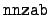= 0, in which case the second line of the file gives the range of targets in terms of the values of 1000*Z + A. The format is:
```    READ(iocins,'(2i6)') nnzab, nnzae
```
A typical line is
```     1 99125
```
In this case the target ranges from a neutron ZA = 1 to a fission fragment ZA = 99125. It is very common to use this option because the standard first line of the `mcfgen.input' file permits the specification of only a single target.

The remaining options are usually not used, and they are given here only for the sake of completeness. These options all depend on the values of flags as described above in the discussion of the first line of the `mcfgen.input' file.

If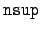= 1 or= 3, the next line of the `mcfgen.input' file gives the range of excitation levels in the format:

```    READ(iocins,'(2e11.4)') elvb, elve
```

If= 2 or= 3, the next line of the `mcfgen.input' file gives the range of temperatures in the format:

```   READ(iocins,'(2e11.4)')  tempb, tempe
```

If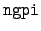= 0, we get the energy bin boundaries from the `mcfgen.input' file. But we first read the number of bin boundaries.

```    READ (iocins, '(i4)') nbounds
READ (iocins, '(6e12.5)') (bounds(i), i = 1, nbounds)
```

If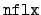= 0, we get the flux from the `mcfgen.input' file. We first read the order of the Legendre expansion lmax, which must be zero. We then read nflux, the number of (energy, flux) entries. Finally, we read the (energy, flux) pairs.

```    READ (iocins, '(i4)') lmax
If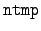= 0, we get the temperatures from the `mcfgen.input' file.
```    READ (iocins, '(i4, 68x, i1)') numtemp